### AND Function Examples in Excel & Google Sheets

This Tutorial demonstrates how to use the Excel AND Function in Excel to test if multiple criteria are all true.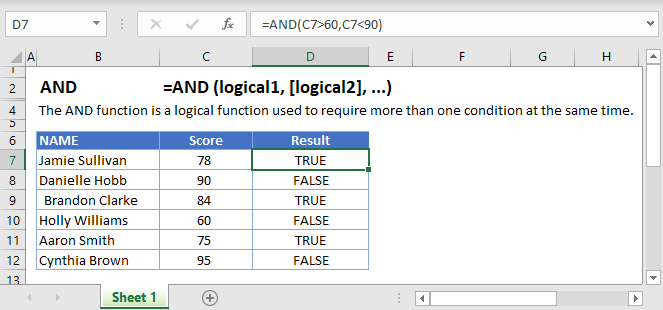## AND Function Overview

The AND Function Checks whether all conditions are met. Returns TRUE or FALSE.

To use the AND Excel Worksheet Function, select a cell and type: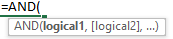(Notice how the formula inputs appear)

## AND function Syntax and inputs:

`=AND(logical1,logical2)`

logical1 – Logical expressions. Example: A1 > 4.

# What is the AND Function?

AND is one of Excel’s logical functions. It evaluates several expressions, and returns TRUE if ALL of the conditions are true. AND can evaluate up to 255 expressions.

AutoMacro - VBA Code Generator

## How to Use the AND Function

Use the Excel AND Function like this:

`=AND(1 = 1, 2 = 2)`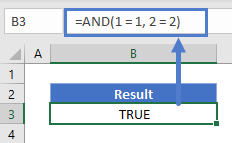Since both of these expressions are true, AND will return TRUE.

However if you used the following:

`=AND(1 = 1, 2 = 1)`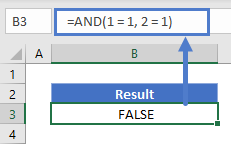In this case AND would return FALSE. Although the first expression is true, the second isn’t.

Note that numerical values alone are counted as TRUE, except zero, which is counted as FALSE. So this formula would return true:

`=AND(1, 2, 3)`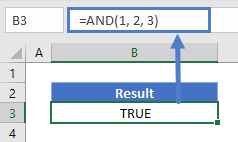But this one would return FALSE:

`=AND(1-1, 2)`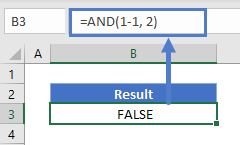This is because 1-1 evaluates to 0, which AND interprets as FALSE.

## Comparing Text Values

Text comparisons with the AND Function are not case-sensitive. So the following formula returns TRUE:

`=AND("Automate" = "automate", "Excel" = "excel")`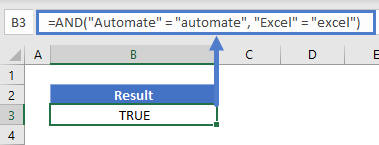Also, AND does not support wildcards. This formula returns FALSE:

`=AND("Auto*" = "automate", "Ex*"="excel")`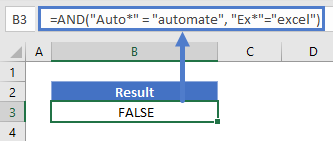This is because AND is literally comparing “Auto*” with “Automate”, which don’t match.

Unlike numbers, text strings alone (when not part of a comparison) are not counted as TRUE – they will return a #VALUE! error.

## Comparing Numbers

You have Excel’s usual range of comparison operators at your disposal when comparing numbers with AND. These are: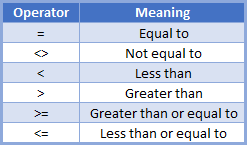## Finding Values Within a Given Range

One handy usage of AND is to locate values that fall within a given range. See the following example:

`=AND(D3>=300, D3<=600)`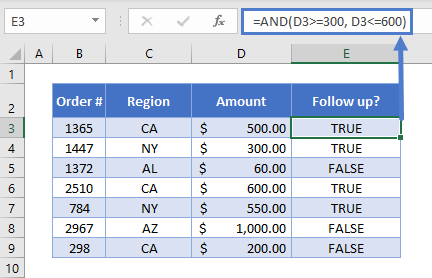Here we have a series of orders, but we need to follow up on all orders between \$300 and \$600, maybe as part of our customer service initiative. So in our AND function, we’ve defined two expressions: D3>=300 and D3<=600.

## Using AND with Other Logical Operators

You can combine AND with any of Excel’s other logical operators, such as OR<<link>>, NOT<<link>>, and XOR<<link>>.

Here’s an example of how you might combine AND with OR. If we have a list of movies, and we want to identify movies released after 1985 that were directed by either Steven Spielberg or Tim Burton, we could use this formula:

`=AND(C3>1985,OR(D3="Steven Spielberg",D3="Tim Burton"))`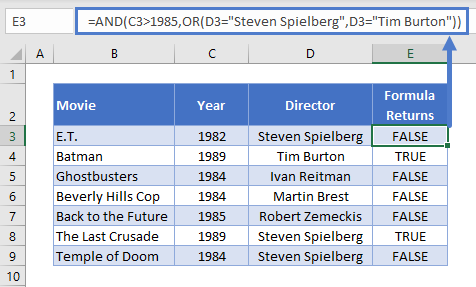Note that whenever you combine logical operators, Excel will evaluate them from the inside-out. So here, it will evaluate the OR statement first, and use the TRUE or FALSE value that OR returns when evaluating the AND Function.

## Using AND with IF

AND is most commonly used as part of a logical test in an IF statement.

Use it like this:

`=IF(AND(C4="CA", D4>300),"Yes", "No")`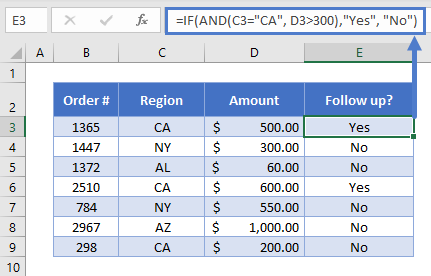This time we want to follow up on all orders from California with a value of \$300 or greater – so that’s what we’ve put in the AND function.

After the AND, we supply IF with two return values. The first for when our AND Function returns TRUE (in this case, we return “Yes”), and the second for when it returns FALSE (we return “No”).

Read more on the main page on the Excel IF Function <<link>>.

## AND in Google Sheets

The AND Function works exactly the same in Google Sheets as in Excel: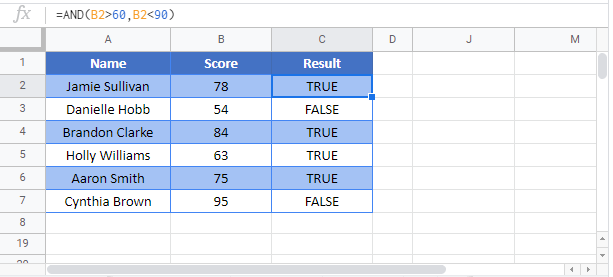## Additional Notes

Use the AND Function to simultaneously test multiple conditions. Each condition must be a logical expression (ex: a1 >5), a reference to a cell containing TRUE or FALSE, or an array containing all logical values. If all conditions are TRUE, the formula returns TRUE, otherwise it returns FALSE.

AND is a logical function and is generally used in conjunction with another logical function, IF:
The IF Function does one thing if a condition is TRUE and does another if a condition is FALSE. Often when using the AND Function you will “nest” it within an IF Formula.

You may also want to use these other logical functions:
The OR Function tests if one or more conditions are met.
The XOR Function tests if one and only one condition is met.

## AND Examples in VBAYou can also use the AND function in VBA. Type:
`Application.Worksheetfunction.And(logical1,logical2)`
For the function arguments, you can either enter them directly into the function, or define variables to use instead.

Return to the List of all Functions in Excel

## Excel Practice Worksheet

Practice Excel functions and formulas with our 100% free practice worksheets!

• Automatically Graded Exercises
• Learn Excel, Inside Excel!